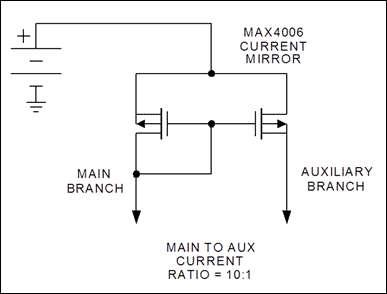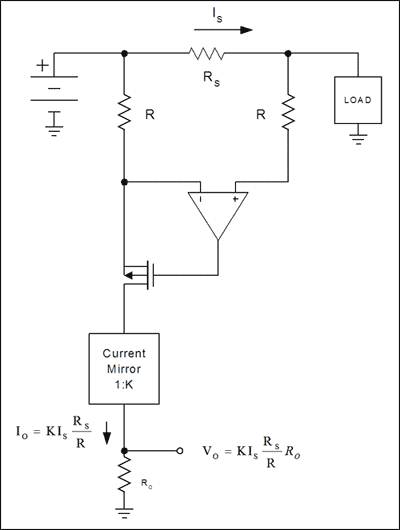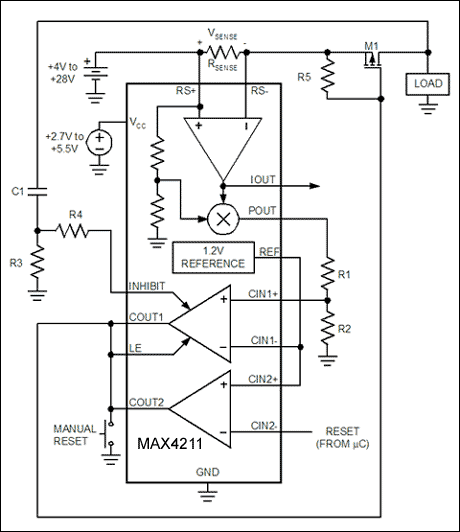# Power-Sensing Circuit Breaker Breaks New Ground

### Abstract

Most circuit breakers sense current. Fuses, for example, protect a circuit by blocking the current when it exceeds a limit. While current-sensing circuit breakers work well for constant DC or RMS supply voltages, power sensing is safer for a variable supply voltage such as the battery in a notebook computer. This article shows the method and benefits of using a power-sensing approach rather than a current-sensing approach to implement a circuit breaker.

### Introduction

The main supply voltage in a notebook computer drops when the wall cube is unplugged. This happens because battery voltage is generally lower than that from a wall cube. Even the voltage of a Li+ battery changes from 4.1V when fully charged to less than 3V when nearly discharged. Thus, a power-sensing circuit breaker is preferable for systems like notebook computers, in which power is limited and the voltage source is not constant.

### Theory of Operation for a Power-Sensing Circuit

Power delivered to a load is defined as the load voltage multiplied by the load current. A power-monitoring integrated circuit, therefore, must include a current-sensing circuit with voltage output and an analog multiplier. The high-side current sensor provides an output voltage proportional to load current, which is multiplied by the load voltage to obtain an output voltage proportional to load power (Figure 1).Figure 1a. A basic power sensor includes a current sensor that measures current and produces a proportional voltage, which is multiplied by the load voltage to obtain a voltage output proportional to load power. The low-cost MAX4210 all-silicon power sensor.Figure 1b. A basic power sensor integrates the current sensor and analog multiplier.

### Silicon High-Side Current-Sense Amplifiers

As Table 1 shows, you can implement current-sensing circuit-breaker circuits in a number of ways. Fuses, resettable fuses, and bimetallic strips are electro-thermal types. Each circuit design places in series with the load a material of known resistance, which heats up as current passes through it. As the current reaches an unsafe (trip) level, the circuit is broken either by melting the material (fuse), increasing the resistance dramatically (resettable fuse), or by heat that causes metal to bend and open a switch (bimetallic strip). The operation of an electromagnetic circuit breaker is similar to that of a bimetallic-strip breaker, except that the mechanical actuation is produced by an electromagnet instead of heat.

Table 1. Comparison of Current-Sensing Technologies

 Type Principle Accuracy Complexity Cost Comment Current switches Fuse Thermal Poor Simple Very low Not resettable Resettable Fuse (PTC) Thermal Poor Simple Very low Circuit breaker (bimetallic strip) Thermal Poor Simple Low Bulky mechanical device Circuit breaker (electromagnetic) Electromagnetic Poor Simple Low Bulky mechanical device Current sensors Current transformer Magnetic Good* Medium to high Medium to high Bulky transformer, AC signal only Hall-effect Magnetic Good* Medium to high High Non-contact magnetic field sensor Giant magnetoresistance (GMR) Magnetic Good* Medium to high High Similar to hall-effect but better sensitivity. Integrated circuit-current mirror (MAX4006) Resistor Good Simple Low Wide dynamic range, low-current capability Integrated circuit (MAX4210) Resistor Good Simple Low Immune to magnetic interference
* Generally requires calibration for good accuracy.

The four current-switch circuit breakers mentioned in the table above do not provide precision current sensing and their trip-point accuracy is poor. In contrast, the current sensors offer better accuracy in sensing and measuring current; each can easily be designed as a circuit breaker.

The current transformer, although generally bulky and complex, is a type of current sensor suitable for AC signals only. It is basically a transformer whose primary is in series with the power source and whose secondary provides a voltage output proportional to the current.

Hall-effect and giant magnetoresistance (GMR) sensors are actually magnetic field sensors. Because any current-carrying wire or PCB trace produces a magnetic field, magnetic sensors are useful for sensing current. They also provide galvanic isolation because they make no contact with the current-carrying conductor. The sensor's output voltage depends not only on the carrying current, but also on the physical location of the current-carrying PCB trace with respect to the sensor. To achieve good accuracy, therefore, Hall-effect and GMR sensors generally require calibration, which increases the circuit's complexity and cost. These types of sensors are also susceptible to magnetic interference from adjacent circuitry.

The current mirror is another way to measure current and consists of two matching transistors of different sizes, connected in a current-mirror configuration (Figure 2). The auxiliary branch mirrors the main branch current by, for example, a ratio of 10:1. Thus, current in the main branch can be measured by a proportional current in the auxiliary branch. The current-mirror sensing technique offers a wide dynamic range (up to 6 decades), but is generally limited to low-current applications.Figure 2. A current mirror in the MAX4006 high-side current monitor measures current over six decades of dynamic range. The current is limited to a maximum of 10mA.

For a current mirror to work, the MOSFET must operate in the saturation region. The voltages across drain and source are typically greater than 1V. When compared with other techniques in which the voltage drop is no more than 0.1V, the 1V drop is excessive. The main current should, therefore, be limited to no more than 10mA as is the case, for example, in measuring the current of a photodiode. The current-mirror technique is good for low-current applications, but is not power efficient for high-current, low-voltage applications involving batteries.

For DC applications such as a battery, the simplest way to monitor current is by exploiting Ohm's Law: V = IR. That is, the voltage across a sensing resistor is linearly proportional to the current passing through it. Resistor-based current sensors generally require an amplifier to provide an output voltage to the external circuitry. Such current-sensing amplifiers can easily be factory trimmed to achieve accuracies of 1% or better (Figure 3). This type of circuit is called a high-side current-sensing amplifier, because it measures current at the voltage source without interfering with the load's ground path.Figure 3. This high-side current-sensing amplifier outputs a voltage proportional to the load current.

The high-side current-sense amplifier places a current-sensing resistor between the battery (voltage-source terminal) and load. That arrangement avoids extraneous resistance in the ground plane, greatly simplifies the layout, and generally improves the overall circuit performance. Current passing through the sensing resistor, RS, develops a voltage drop across that resistor. Sensed by the op amp, this voltage drives the MOSFET transistor to sink current passing through R. The voltage drop across R is equal to the voltage drop across the sensing resistor. Hence:

KISRS = IOR,
IO = KIS(RS/R), and
VO = KIS(RS/R)RO.

The sensor output current is proportional to the load current. Typically, a current mirror is included to increase the output current by a factor of K. If you require a voltage output, convert the current to voltage by placing an output resistor, RO, between the current output and ground. Resistors R and RO can easily be factory-trimmed to achieve a current-sensing accuracy of 1% or better.

### Power Monitor

Now that your design measures current with an accurate and low-cost current sensor, you can obtain the power measurement by multiplying the current times the voltage. A low-cost, all-silicon power sensor consists of an integrated current sensor and analog multiplier. (See Figure 1b above.) The multiplier operates in the first quadrant, that is, both input and output are positive voltages. Only a single supply voltage is required. Like the current sensor, the analog multiplier can also be factory-trimmed to achieve good accuracy.

The MAX4210 power-monitor IC, for example, is designed to monitor the battery in a notebook computer. The power sensor's common-mode voltage range (4V to 28V) accommodates a variety of battery voltages. To measure current, a sense resistor is inserted in the path between power source (battery) and load. The current-sense amplifier then feeds a voltage proportional to load current to one input of the analog multiplier. The other multiplier input connects to a voltage-divider connected to the load. (Load voltage must be reduced by a divider, because the multiplier's maximum input voltage is 1V.) Multiplying these two voltages produces an output voltage proportional to load power.

Factory trimming is required to achieve the desired accuracy for the power measurement. Figure 4 shows typical power-measurement accuracy vs. sense voltage for the MAX4210 after factory trimming. For VSENSE between 50mV and 150mV, the error is less than 1%. For sense voltages below 50mV, the measurement error increases due to input offset in the current-sense amplifier. The sense resistor is, therefore, chosen to ensure that the voltage drop across it is between 50mV and 150mV at maximum current. If the maximum anticipated load current is 10A, for example, choose a 10mΩ sense resistor which produces a full-scale voltage of 100mV.Figure 4. The power-measurement error of the MAX4210 high-side power and current monitor increases at low-sense voltages, due to input offset in the current-sense amplifier. For sense voltages greater than 50mV, the error is better than 1%.

### Electronic Power-Sensing Circuit Breaker

In systems for which the supply voltages vary or the supply is power limited, or both, a circuit breaker that senses overpower faults offers better protection than one that senses current faults only. Such systems include notebook computers, smart batteries, and high-reliability power supplies. Power-sensing ICs such as the MAX4211 are designed for circuit-fault protection.

The power-sensing circuit breaker, useful for protecting batteries from short circuits and overpower faults, blocks current to the load when the breaker detects an overpower fault (Figure 5). Once a fault is detected, the MOSFET, M1, turns off and stays off until the manual-reset button is pressed or a logic-high is applied to the CIN2- input. You can also reset the circuit breaker by cycling the input power, which causes the LE pin to go low and unlatch the comparator output, OUT1. The RC network, R3-R4-C1, connected to the comparator prevents false transitions during a voltage transient.Figure 5. This solid-state, power-sensing circuit breaker shuts off current to the load in response to an overpower fault. Pressing the manual reset button or applying a logic-high to CIN2- resets the circuit breaker. The comparator INHIBIT input prevents false overpower alarms by temporarily suspending itself when a voltage transient occurs.

### Conclusion

For battery applications in which terminal voltage varies as the battery discharges, power monitoring is preferable to current monitoring. Power monitoring is also safer. Modern ICs integrate most of the components necessary to make a low-cost, high-performance, all-silicon power sensor. Such ICs are factory-trimmed to achieve better than 1% accuracy. Combining their internal comparators and reference with an external MOSFET switch forms a device not easily realizable until now—a simple, power-sensing circuit breaker like the MAX4210.

### Related Parts

The MAX4172 and MAX4211 are related parts. For more information, please see the MAX4172 and MAX4211EEVKIT and data sheets.